Diffraction

If a wave is incident on a grating which has a sinusoidal transmittance profile, three exit waves are produced. This is the case whether it be electromagnetic waves, electrons, light, or even water waves. This effect is known as diffraction.

Understanding diffraction provides an interesting insight to how information about an object is encoded into light which is scattered by it. Huygens model of wave propagation, though good for explaining reflection and refraction, does not give a very good insight into diffraction. A very interesting alternative method is that of fourier optics. I hasten to add, before you click your 'Back' button, that very little mathematics is required.

How Diffraction Works

Diffraction can be better understood with a couple of diagrams and a little trigonometry. The following description uses light waves but it is equally applicable to other waves.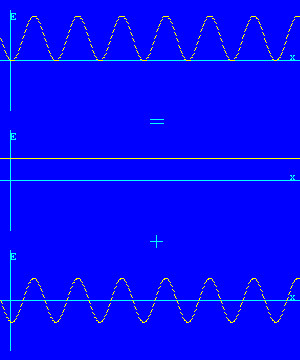The top graph on the left shows the E field of the light across the exit side of the grating. You have to imagine that it is oscillating up and down at the frequency of the light (about 4x1014 times a second). It is the input beam multiplied by the sinusoidal transmittance profile of the grating.

The top graph can be broken down into two sum components. These are shown in the middle and bottom graphs.

The first component (middle graph) is just an attenuated version of the input wave. It therefore exits the grating travelling in the same direction as the input beam.

The second component (bottom graph) is a standing wave. A standing wave is made up of two waves traveling in opposite directions. The period of this standing wave is that of the grating (d). The period of the light cannot change though. The only way that this can be so, is by the two waves traveling away from the grating at equal and opposite angles.

So the top graph represents three exit beams; one straight through, and two at equal but opposite angles.

To see what the exit angles will be, a little trigonometry is required, as shown in the following diagram: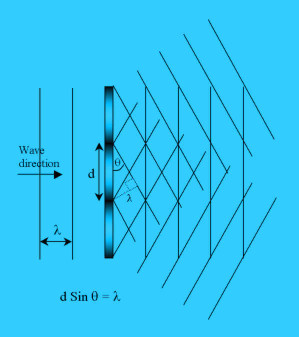The diagram on the left shows a beam of wavelength lambda approaching a sinusoidal grating at a normal to it. It leaves the grating as three beams as explained above.

The angles of the beams have to be such that the standing wave across the grating has the same period (d) as the grating, as can be seen in the diagram.

From the construction, it can be seen that the grating period (d) multiplied by the sin of the angle between a wavefront and the grating (θ) is equal to the wavelength of the light (λ). The angle between an exit beam and the grating is 90o-(180o-90o-θ) = θ.

This gives the relationship d Sin θ = λ.

Practical Investigation

Further insight can be gained by looking at the diffraction patterns created by simple objects. To make the diffraction patterns as simple as possible, monochromatic coherent light should be used. A low powered laser is ideal for this.

All that is required is a helium/neon laser or visible laser diode, a transmission type object and a screen. The screen can be a sheet of white card. A laser pointer would probably work although I have not tried it.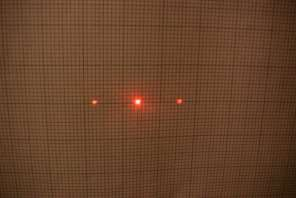From the above theory, you should be able to guess what object made this diffraction pattern. The object was a sinusoidal grating made using the same technique used for a simple transmission hologram, but with an unmodulated object beam. The sinusoidal interference pattern crated by the two beams was recorded on the film.

In holographic terminology, one side spot is the real image of the object beam and the other is the pseudoscopic image.

The distance from the grating to the screen was 410mm and the spots are 23mm apart. Therefore the deflection angle θ = tan-1(23/410) = 3.2o. The laser was a helium/neon type, with a wavelength of 633nm. Using the formula d Sin θ = λ, the grating period d = 630nm / sin 3.2 = 11μm.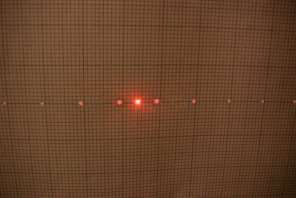How can this diffraction pattern be explained by the theory developed so far? Well the middle three spots could have been created by the sinusoidal grating used for the previous pattern. The next pair of spots from the centre could have been produced by a sinusoidal grating with one third the period and the next pair by one fifth the period.

This is where it gets interesting. This is the diffraction pattern produced by a square wave grating with equal mark to space ratio. The square wave consists of a fundamental frequency and an infinite number of odd harmonics, each producing a pair of spots in the diffraction pattern. So a diffraction pattern is a fourier transform of the object. Each pair of spots represents a spacial frequency of the object.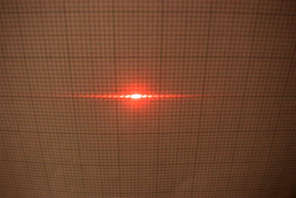This is the diffraction pattern created by a vertical slit. The slit object was simply two Stanley knife blades attached to a magnet.

The important points to note are that the diffraction pattern of a vertical slit causes only horizontal deflection in the diffraction pattern and that the pattern consists of a central bright line and then many shorter 'dashes' fading out in either direction. The fourier transform of a 'top hat' function (the slit) is a sinc function. Because you can only see intensity variations, the diffraction pattern resembles a sinc2 function.

When the slit is made narrower, the dashes get longer and move further apart as in the following two pictures.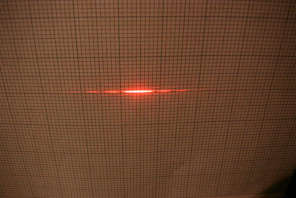Rotating the slit, rotates the diffraction pattern as shown below. In the left hand picture, the slit is at 45o. In the right hand picture the slit is horizontal.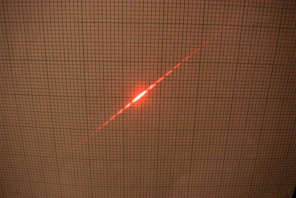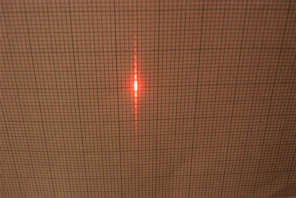Altering the Diffraction Pattern (Spacial Filtering)The above picture is the diffraction pattern of a fine wire mesh. The mesh is constructed from horizontal and vertical wires, equally spaced apart. The diffraction pattern therefore mainly contains information in the horizontal and vertical directions. There are spots for all harmonics of the mesh period because the mark to space ratio is not 1:1. The mesh to screen distance was 1.17 meters. The largest squares on the grid are 20mm wide, so there are about 2.5 spots per cm. I have worked out that the wire spacing (period) in the mesh is about 0.18mm.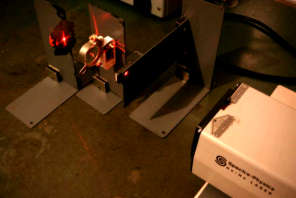This is a simple setup for spacial filtering.

A lens produces a diffraction pattern in the back focal plane. An image is produced on a distant screen. The diffraction pattern can then be altered using masks and the result on the image observed.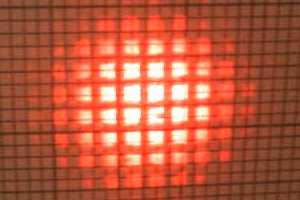This is an image of the mesh. The diffraction pattern has not been altered in any way.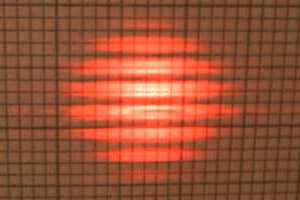This is the image after the horizontal spacial frequency information has been removed from the diffraction plane using a pair of blades as a mask.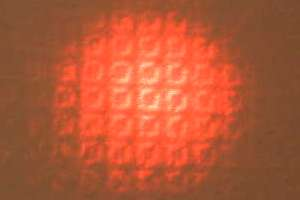This is the image after the low spacial frequencies have been removed. The squares are no longer 'filled in' fully.

This is the result of removing the central portion of the diffraction pattern with a mask.

Imaging Phase Objects by Spacial Filtering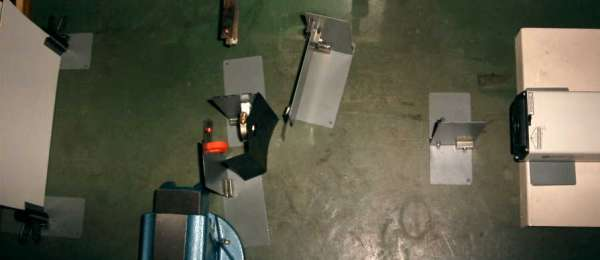A fingerprint is almost invisible to the naked eye. This is because it is a phase object. It causes phase shifts in the light due to the thickness of the oil, but does not cause much intensity variation.

A phase object causes diffraction in a similar way to an amplitude object. It can be rendered visible by using a high pass spacial filter. All that is required is a 'stop' in the center of the diffraction pattern to remove the zero and low spacial frequencies.

My spacial filter lash up is shown in the picture above. The laser is on the right and the screen on the left. The grey things are home made magnetic stands. Immediately to the left of the laser is a diverging lens so that the laser beam illuminates the whole object. The next stand to the left holds the object which is a piece of film with a finger print on it. Next there is a card mask to block light which would otherwise pass around the outside of the next lens. The lens immediately to the left of the card mask produces a diffraction pattern of the fingerprint in its back focal plane. The center of the diffraction pattern is removed by a small spot of Blu-Tack attached to a piece of clear plastic film. The orange thing is just a magnet holding the film to the stand. The image is then formed on the screen on the far left.This is the image of the fingerprint after spacial filtering. The background appears black because the photograph was taken with the room lights off to improve the contrast.

This type of filtering is called the dark field technique.

Information about diffraction as it applies to individual photons is on the Young's Slits page. The Young's slits single photon diffraction experiment is also provided live online (not a simulation). For the experiment, you will require Java and some patience.